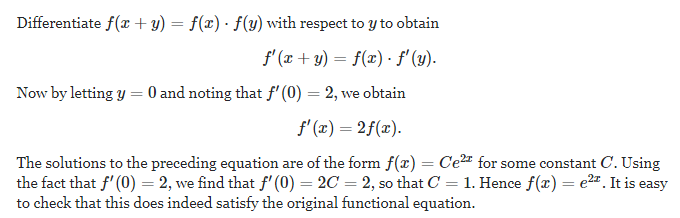# p { margin-bottom: 0.25cm; line-height: 120%; }A function f:R-->R,satifies the equation f(x+y)=f(x)(y) for all x,yєR and f(x) is not equal to 0 for any x in R.If x be differentiable at x=0 and f’(0)=2,show that for all xєR,f’(x)=2f(x)Anish Singhal